# Steiner trees and soap bubbles

March 11, 2020. What do railway lines, honeycomb, and soap bubbles have in common? Read on to find out! A self-contained tutorial on the geometry and topology of minimal networks, now with extra goodness on Plateau’s laws and three-dimensional foams.

### Contents

1. Introduction
2. Triangles
3. Graphs
4. Soap bubbles

## 1. Introduction

Suppose we want to join up three towns ($A$, $B$ and $C$) by rail. If I can use this railway to travel from one town to any other, we say that the rail network is connected. Two simple examples of connected networks are shown below.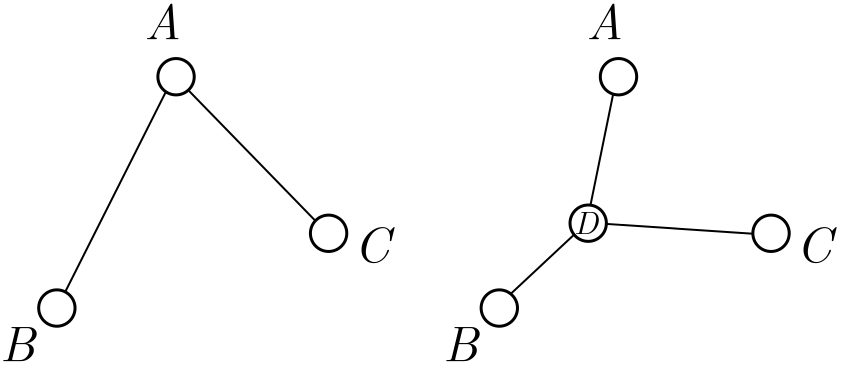Figure 1. Rail networks (triangle and trident) connecting three towns.

Building railways is expensive, since we not only need to design and build the rail itself, but acquire the land beneath it. In contrast, stations are cheap: we just slap together some sidings, a platform, and a bench or two, and voila. To minimise cost, we should make the total length of the rail network as short as possible, adding extra stations if they help us do this. The resulting layout is called the minimal network or Steiner tree. (We will see where the “tree” comes from later.)

We can consider the same problem for $n$ stations, and find the rail networks of smallest total length which connect them. Although finding the exact minimal network is difficult in general, we can derive some beautiful results about their geometry and layout. We’ll step through the reasoning slowly, starting with case of three cities. The only prerequisites will be trigonometry, algebra, and some mathematical pluck.

Applications. Whenever you want to optimise the design of a network, chances are that the minimal networks we’ll consider, or a variant, are not too far away. Examples range from railways to computer chip design, telecom networks to the classification of life itself. This is definitely real-world mathematics!

Exercise 1 (choosing sides). Suppose $A$, $B$ and $C$ are separated by distances $AB = c$, $AC = b$ and $BC = a$. A triangular network consists of two sides of the triangle. Which ones should we choose?

Exercise 2 (triangle or trident). In Figure 1, we have two networks connecting the same towns: a triangular network, and a trident-shaped network with a hub station $D$ in the middle. Which is shorter? See if you can do better than either.

Hint. Measure lengths on the screen with a ruler. Simple but it works!

## 2. Triangles

The three-town problem is already surprising. The simplest network consists of two sides of the triangle, but it is not minimal; to minimise length, a trident-shaped network, with a new hub station $D$ in the centre, is optimal. The best place to put the hub station is called the Fermat-Torricelli point. For the rest of the post, we will only worry about when we should build a hub, rather than where it goes exactly. This will save us some hard trigonometry! The one exception will be an important special case: the equilateral triangle.

### 2.1. Equilateral triangles

Suppose that the towns $A$, $B$ and $C$ sit on the corners of an equilateral triangle of side length $d$. The triangular network has total length $L_\Lambda = 2d$.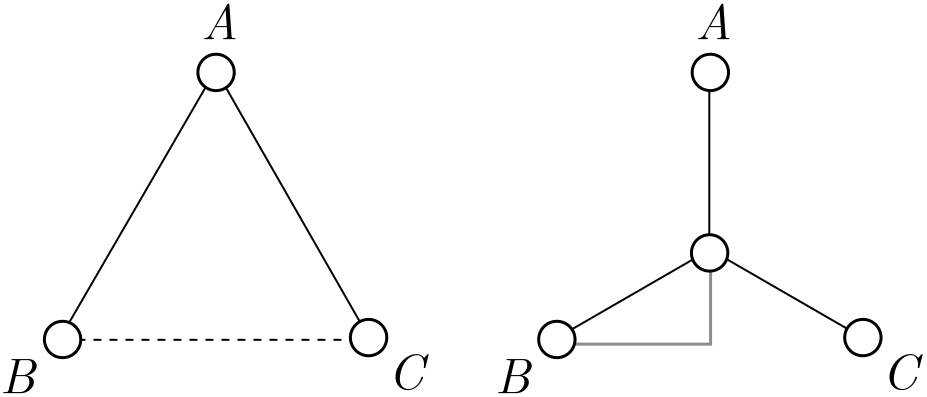Figure 2. Rail networks on an equilateral triangle.

It seems plausible that the trident network does better, but instead of checking with a ruler, let’s do some basic trigonometry.

Exercise 3 (equilateral trident). Show that the length of the trident network, with a hub in the centre of the triangle, is

$L_{\text{Y}} = \sqrt{3}d.$

Since $\sqrt{3} \approx 1.7 < 2$, the trident is indeed shorter than the triangle.

Hint. Use the grey triangle in Figure 2.

You might guess that the best place to put the hub station $D$ is right right in the centre, and in fact, we can prove this from symmetry. First of all, draw an axis of symmetry of the triangle, represented by the red line in Figure 3.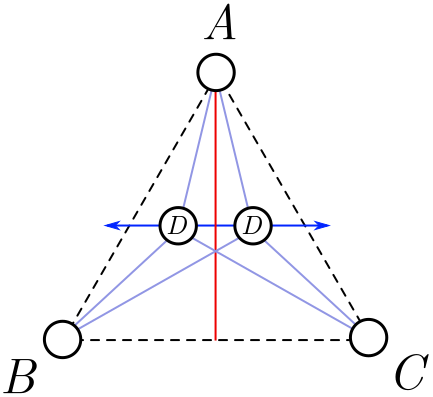Figure 3. Wiggling the hub around an axis of symmetry.

We are going to wiggle the hub side to side around this axis, along the dark blue line in Figure 3. From left-right symmetry, the length must either be a minimum or a maximum on the axis itself. Since the total nework length gets very large when we take the hub outside the triangle, it must be a minimum. So, for a minimal network, we must place $D$ on the red line.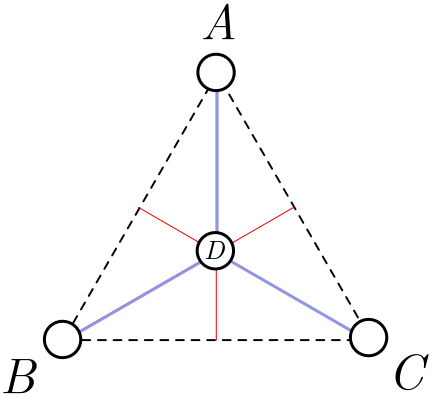Figure 4. The minimal network on an equilateral triangle.

But there are axes of symmetry associated with $B$ and $C$ as well, and all three intersect at the centre of the triangle. Since $D$ should lie on each of these lines, it must lie at the centre! This completes our proof. The minimal network is depicted in Figure 4.

### 2.2. Deforming the triangle

We are now going to take our solution to the equilateral triangle and slowly deform it. In other words, we imagine slowly moving the corners of the triangle so it is no longer equilateral. What will happen to the optimal position of the hub $D$? Since everything is being continuously modified, the position of the hub should change continuously as well. In Figure 5, the paths of the corners are depicted in purple, and the corresponding continuous change of hub in green.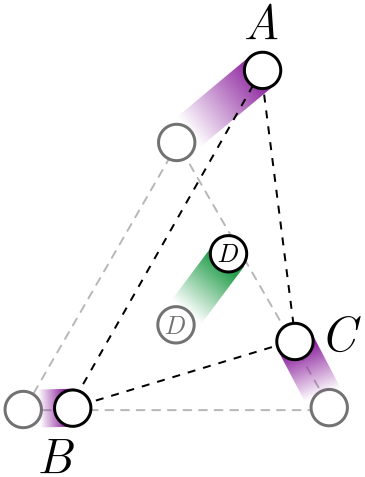Figure 5. The optimal hub position shifts as we deform the corners.

Since the hub position changes continuously, it should stay inside the triangle for small deformations of the corners. But for triangles which are very far from equilateral, the hub might intersect one of the corners, and our trident network becomes a simpler triangular network, formed from two sides of the triangle.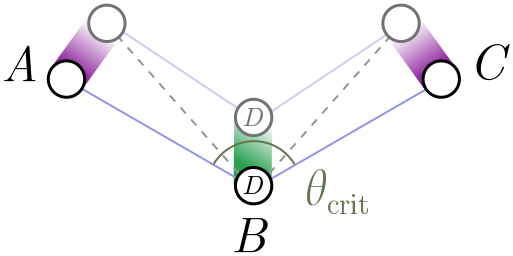Figure 6. At some critical angle, the trident network becomes triangular.

We picture this in Figure 6 above. In this diagram, $B$ remains fixed in position, but $A$ and $C$ slowly lower and open out the angle of the triangle, with the optimal hub $D$ moving down as they do so. At some critical angle $\theta_\text{crit}$, it will coincide with $B$. The question is: what is the angle?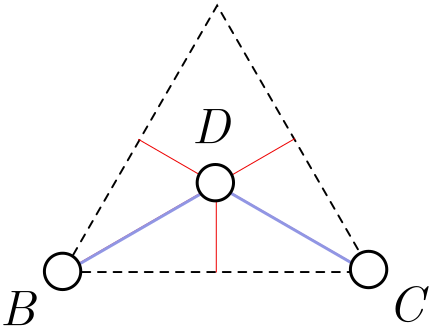Figure 7. Removing a corner city removes a leg from the equilateral trident.

It turns out this critical angle is $\theta_\text{crit} = 120^\circ$. Although we won’t provide a watertight proof, we can give a plausibility argument. Let’s return to the equilateral triangle. Instead of adding a hub in the middle, suppose that $D$ is in fact a fourth city fixed in place. Clearly, the solution in Figure 4 is still optimal, since if we could add more hubs to reduce the total length, we could add more hubs to improve the network for the equilateral triangle! If I now remove any of the corner cities $A$, $B$ or $C$, the optimal network simply removes the corresponding leg of the trident (Figure 7).

Exercise 4 (cutting corners). Suppose that in Figure 7, we can add a new hub $E$ which reduces the length of the network. Explain how adding $E$ could reduce the length of the network in Figure 7, and thereby improve our solution for the equilateral triangle.

Note. This is an example of a proof by contradiction. To show something is false, we assume it is true and use it to derive a contradiction with known facts. We then reason backwards to conclude that it cannot be true!

Exercise 5 (critical isosceles). The argument above really only establishes that $\theta_\text{crit} \leq 120^\circ$. It is possible, in principle, that the triangular network becomes shorter at some angle $\theta_\text{crit} < 120^\circ$. In this exercise, we will show for an isosceles triangle that this is not the case.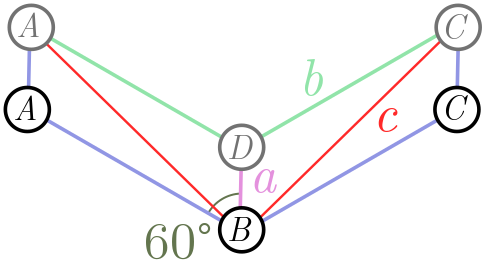Figure 8. Critical angle on an isosceles triangle.

Figure 8 shows the triangular network (blue lines) $ABC$, forming an angle of $120^\circ$. We now raise the two nodes $A$ and $B$ at the end, so that the angle $ABC$ is less than $120^\circ$. You can prove that the green and purple lines are shorter than the red lines, so that an interior hub $D$ yields a shorter network.

(a) Show using the law of cosines (or otherwise) that

$c^2 = a^2 + b^2 + ab.$

(b) From part (a), show that

$a + 2b < 2c.$

(b) Conclude that for an isosceles triangle $ABC$, the critical angle is $\theta_\text{crit} = 120^\circ$.

### 2.3. Hubs and spokes

All this work with triangles pays off with a powerful conclusion for minimal networks connecting any number of cities:

In any minimal network, all hubs have three incoming legs separated by angles of $120^\circ$.

The argument is beautiful and simple.

Proof. Our first step is to show that it is impossible for a hub to have edges separated by less than $120^\circ$. Suppose we have cities $A_1, A_2, \ldots, A_n$ connected by a minimal rail network. Also suppose there is a hub station $H$ with incoming rail lines separated by less than $\theta_\text{crit} = 120^\circ$, as on the left in Figure 11. There may be other incoming lines (the horizontal lines in Figure 11), but these will play no role in our proof and can be ignored.

We can build two new stations on these outgoing legs, $h_1$ and $h_2$, which are (for simplicity) the same distance from $H$.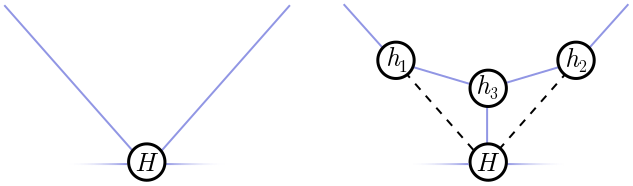Figure 9. Left: A hub with incoming angle less than 120°. Right: A shorter network.

It’s easy to see that every angle in this triangle is less than $120^\circ$, since the angles at $h_1$ and $h_2$ are at most $90^\circ$, and we’ve assume the angle at $H$ is less than $120^\circ$. Thus, from our work in the previous section, we know that the minimal network connecting $h_1$, $h_2$ and $H$ is not the triangle we have drawn, but a trident with another hub $h_3$ in the middle! This strictly decreases the length of the network (shown right in Figure 9), and hence, our original network must not have been minimal. There’s our contradiction!

So, we know that any hub must have spokes separated by at least $120^\circ$. How do we know that there are three, separated by exactly $120^\circ$? This is very simple. Suppose two lines enter $H$, separated by more than $120^\circ$. Then there can only be two incoming edges, joining $H$ to some cities $A$ and $B$, since any additional lines would have to be closer than $120^\circ$ to one of these lines, given the result we just proved. So, we have the situation depicted on the left of Figure 10:Figure 10. Left: A hub with incoming angle greater than 120°. Right: A shorter network.

Hopefully you can see what goes wrong. If there is a “kink” in the line, with separating angle $\theta \neq 180^\circ$, then we can obtain a shorter network be deleting $H$ and directly connecting $A$ and $B$. Once again, we have a contradiction!

Now, strictly speaking, we can have hubs with only two incoming edges, separated by $180^\circ$. But such a hub is always unnecessary, since all it does is sit on a straight line. In real life, hubs do cost some money (even if it is dramatically less than spokes) so we will never build unnecessary ones! If we always delete these useless hubs, we have the general result advertised above, namely that any hub in a minimal network has three equally spaced spokes.

Exercise 6 (outer rim). Our proof applies to hubs only, but the same style of argument allows you to deduce properties of the cities or fixed nodes $A_1, A_2, \ldots, A_n$. Prove the following properties:

(a) No incoming edges can be separated by less than $120^\circ$.

(b) The number of incoming edges is between $1$ and $3$.

(c) A city has three edges only if it lies at the centre of an equilateral triangle of nodes.

The upshot is that the fixed nodes can have from one to three edges, with three only in special circumstances.

Exercise 7 (easy polygons). Consider cities on the corners of a regular $n$-gon. Show that for $n \geq 6$, the minimal network is just the perimeter with one edge removed.

## 3. Graphs

In this section, we will learn more about the connectivity and layout of minimal networks. The geometry we learned in the last section will be essential!

### 3.1. Trees

In a minimal rail network, how many ways can I get from $A$ to $B$? If the network is connected, then the answer is at least one. That’s the definition of connected! But if the network is minimal, the answer is exactly one. If there is more than one way to get from $A$ to $B$, the network has unnecessary edges and can be pruned to get something shorter. We might worry that pruning redundant paths between $A$ and $B$ could disconnect other cities, but this is never the case. Figure 11 shows why.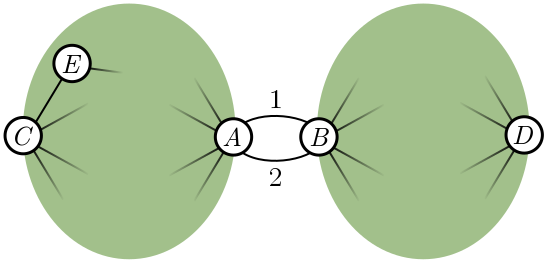Figure 11. Pruning an unnecessary path, e.g. path 2, cannot disconnect a network.

Suppose $A$ and $B$ are connected by two paths, labelled $1$ and $2$. The green blob to the left is all the nodes whose paths to $B$ go through $A$ first, and similarly, nodes on the right connect to $A$ through $B$. If two nodes are in the same blob, such as $C$ and $E$, then pruning path $2$ has no effect on whether they are connected. If two nodes are in different blobs, like $C$ and $D$, they can still reach each other using path $1$. So, pruning redundant paths between $A$ and $B$ does not affect whether other nodes connect.

Exercise 8 (path pruning). Generalise the argument above to account for nodes that lie between $A$ and $B$. More precisely, these are nodes which can connect to either $A$ or $B$ without passing through the other, and schematically lie on paths $1$ or $2$.

Once we have completely pruned the network, there is only a single path connecting any two nodes $A$ and $B$. Such a network is called a tree because of the way it branches. For this reason, minimal networks are more often called Steiner trees. An example is shown in Figure 12.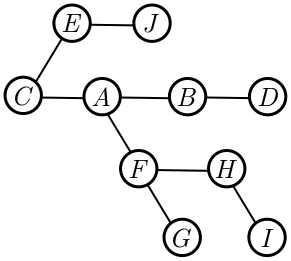Figure 12. A tree network, with unique paths between each node.

Our reasoning shows that minimal networks are trees. The number of nodes in the network is $N = n + h$, where $n$ is the number of fixed nodes and $h$ counts the hubs added to make the network as short as possible. In Figure 12, for instance, we have $N = 10$. The number of edges $E = 9$ is one less than the number of nodes. This is not a coincidence, and for any tree, it turns out that $E = N - 1$. To prove this, we first need to know that (unlike real life), in graph theory, every tree has leaves.

Exercise 9 (trees and leaves). A leaf is a node with a single edge, e.g. the nodes $J$, $D$, $G$, and $I$ in Figure 12. Part (a) will show what we need, namely that every tree has a leaf, and parts (b) and (c) probe this question a little more deeply. To begin, we will choose a node at random (red in Figure 13) and count the number of steps to each other node. Since there is a unique path between two nodes in a tree, this is well-defined.Figure 13. Finding leaves in a tree.

(a) Consider the node or nodes furthest from our selected node (orange nodes in Figure 15). Argue that these must be leaves. Hint. If they are not, what are the distances of the neighbours?

(b) Take the furthest node and now find the node or nodes furthest from it. Argue that these are also leaves, and conclude that every tree has at least two leaves.

(c) Show, using an example, that a tree need not have more than two leaves.

The procedure for finding leaves is illustrated in Figure 15. We start with an arbitrarily chosen node $C$, and calculate distances to all other nodes. The most distance node is $I$, which is indeed a leaf. Measuring from $I$, the most distant node is $J$, which is also a leaf.

We now know that every tree has a leaf. If we remove it, and the single edge connecting it to the rest of the graph, we decrease the number of nodes and edges by $1$, $N \to N- 1$ and $E \to E - 1$. We keep doing this until we are down to a single node. This has no edges at all, and since we decrease by $N-1$ nodes to obtain a single node, decreasing $E$ by the same amount gives $0$. Hence, for trees in general,

$E = N - 1.$

### 3.2. Bounding hubs

If we combine our geometric results from earlier with our result about trees, we can constrain the layout of minimal networks further. Recall that each of the $h$ hubs (once we delete the useless ones) has exactly three edges. Each of the $n$ fixed nodes has at least one edge to ensure it is connected to the rest of the network. Thus, the total number of edges $E$ obeys the inequality

$E \geq \frac{1}{2}\left(n + 3h\right).$

In diagrams,

The factor of $2$ occurs because we are counting edges twice in the leftmost and rightmost expression: each end of an edge is associated with a vertex, and we are counting the contribution at each vertex once. From the previous section, we know that $E = N - 1$. This gives

$\frac{1}{2}\left(n + 3h\right) \leq N - 1 = n +h - 1.$

After some algebra, we find that

$h \leq n - 2.$

The maximum number of hubs $h = n - 2$ is achieved when each fixed point has precisely one edge. In this case, the leaves of the graph are exactly the fixed nodes.

### 3.3. Tinkertoys

Combining the geometry and graph results gives us a simple tool for building minimal networks. For $n$ fixed nodes, pick $h = n-2$ hubs, with spokes emerging at angles of $120^\circ$, and connect them together to form a tree. We call this configuration of hubs and spokes a tinkertoy. It is almost rigid, but we can extend the spokes and legs. For $h = n-2$, the minimal network is always a tinkertoy, but see Exercise 10 for an example of a minimal network which is not.

Let’s illustrate the tinkertoy approach for fixed nodes on a rectangle. There is only one tinkertoy, connecting the closest external nodes in pairs, as shown in Figure 14.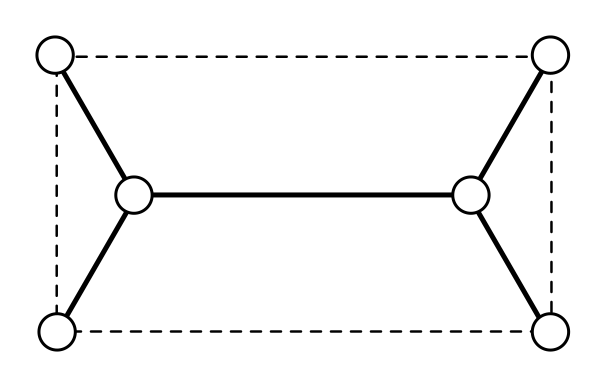Figure 14. The tinkertoy for a rectangle.

The situation for a general quadrilateral is a bit trickier, but can be quickly solved by a computer fiddling with the tinkertoy.

Exercise 10 (minimal network on a rectangle). A rectangle has width $w$ and height $h$, with $w \leq h$. Show that the minimal network has length

$L = w + \sqrt{3}h.$

Exercise 11 (harder polygons). When $h= n-2$, you can use a single tinkertoy, but if $h < n - 2$, you will need multiple tinkertoys. Here we give examples of both.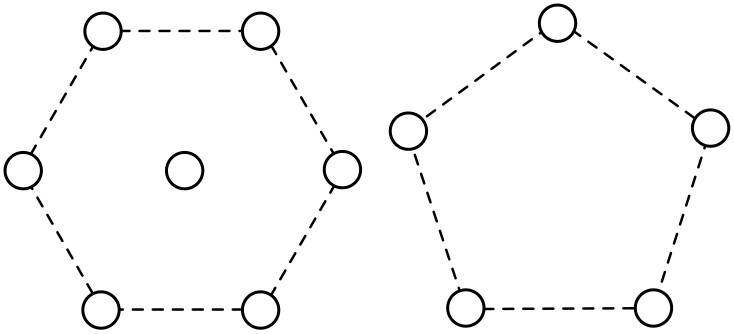Figure 15. Centred hexagon and pentagon.

(a) Find the minimal network connecting a regular hexagon with a node in the centre. (To be clear, this central node is fixed.) You will need multiple tinkertoys!

(b) Draw the tinkertoy for a regular pentagon, and schematically indicate what the minimal network looks like.

For a small number of fixed nodes, tinkertoys are useful. But how useful are they for many nodes? Let’s assume that fiddling with tinkertoys is a quick operation, and we can tell after some fixed time (independent of $n$) whether a particular tinkertoy can connect the fixed nodes. Unlike the cases we’ve look at so far, for large $n$, there are many different tinkertoys to try out. For instance, we show the four different tinkertoys for $h = 6$ (or $n = 8$) in Figure 16.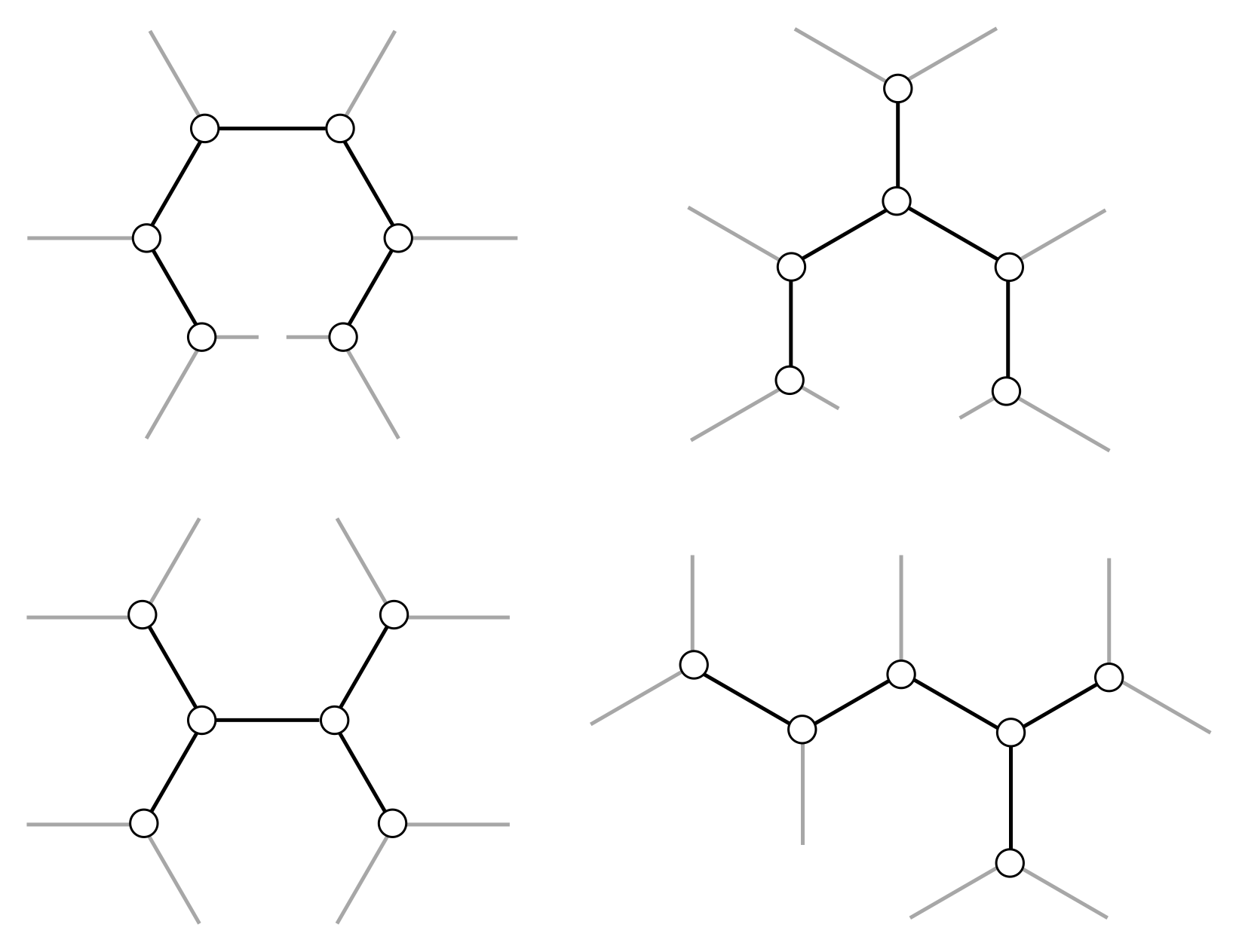Figure 16. A few tinkertoys for h = 6.

In Exercise 12, you’ll prove that there are exponentially many tinkertoys with $h$ hubs as $h$ gets large. A brute force approach, which simply checks each tinkertoy, will take an exponential amount of time, and since exponential get large very quickly, this approach is not computationally feasible for large $h$. Unfortunately, there are no algorithmic shortcuts! You can eliminate some tinkertoys from consideration, but as $n$ gets large, there will always be fixed nodes which require you to try an exponential number. This makes the mathematical solution of the minimal network effectively impossible to compute.

Computer science nerds only. You might be wondering what “effectively impossible to compute” means. Roughly, it means that for $n$ fixed nodes, the running time $t(n)$ for the best known algorithm grows faster than any polynomial in $n$. Let’s pick a polynomial $p(n)$ of degree $k$. As $n$ gets large, only the degree $k$ term $\alpha n^k$ matters, so we can write:

$t(n) \gtrsim \alpha n^k$

for any $k$ and constant $\alpha$, where $\gtrsim$ means “for all $n$ large enough”. This is consistent with our argument from the exponential number of tinkertoys.

The technical term for “effectively impossible to compute” here is NP-hard, meaning computer scientists are pretty darn sure there is no shortcut. While exactly solving Steiner trees is crazily difficult, it turns out to be easy to approximate them, so it lies in PTAS (for “polynomial-time approximation scheme”). We can snatch approximate victory from the jaws of computatonal defeat!

Exercise 12 (counting tinkertoys). Counting the precise number of tinkertoys $T_h$ for any number of hubs $h$ is difficult. But we can cheat in two different ways: show that $T_h$ is greater than some exponentially growing sequence, $S_h$; or use “physicist’s induction”, where we calculate for small $h$ and guess the rest of the sequence.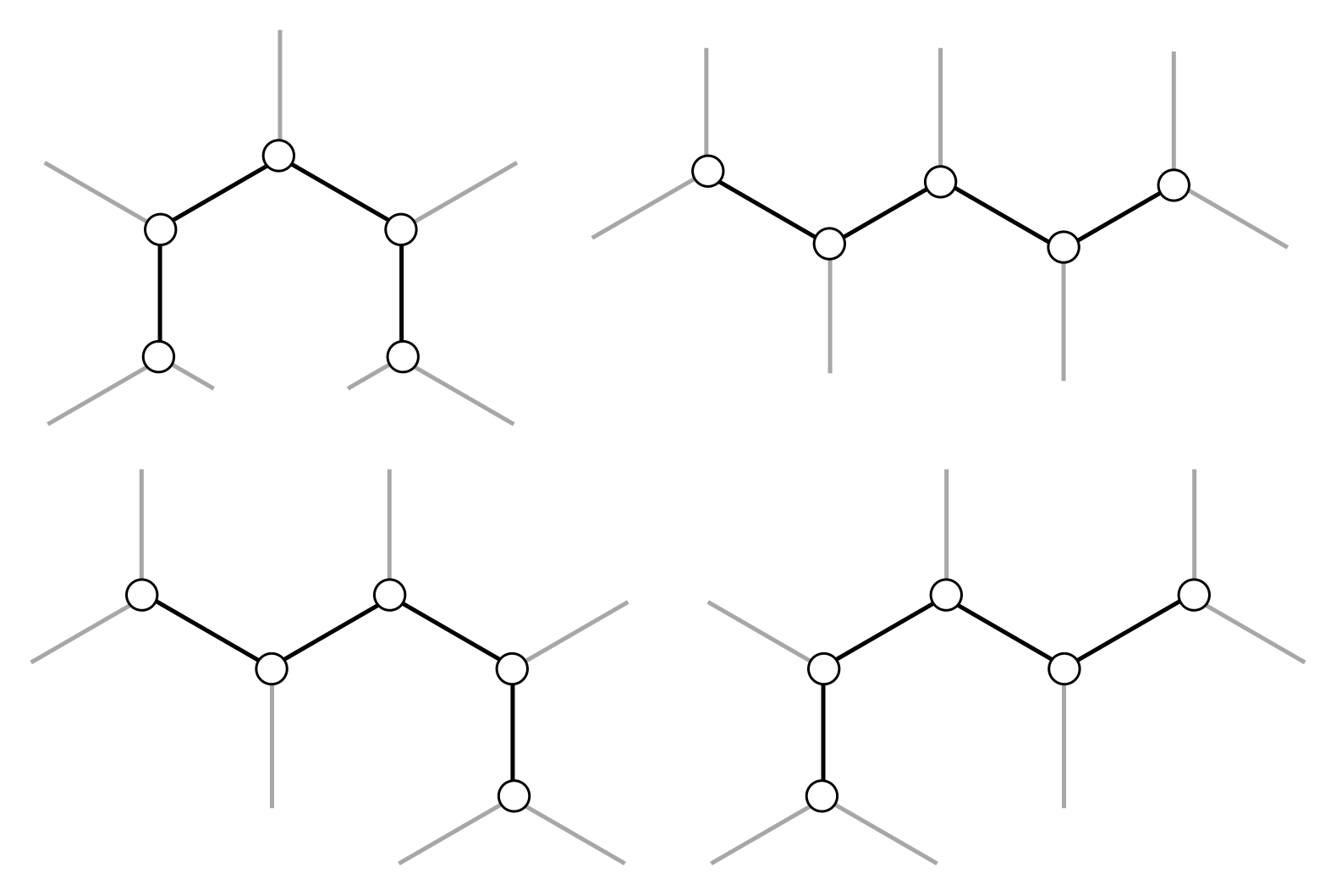Figure 17. Snake tinkertoys for h = 5.

(a) Consider the set of “snake” tinkertoys, where hubs are connected in a single line. Show that, for $h \geq 3$ hubs, there are $S_h = 2^{n-3}$ snake tinkertoys. Since $T_h \geq S_h$, this demonstrates that the total number of tinkertoys grows exponentially.

(b) Calculate the number of tinkertoys from $h = 0$ to $h = 6$. You should be able to find the general sequence $T_h$ by looking for these numbers in the Online Encyclopedia of Integer Sequences. At large $h$, this sequence is indeed exponential, with

$T_h \approx \frac{2^{2n-4}}{\sqrt{\pi}h^{5/2}}.$

### 3.4. Historical notes

French mathematician Pierre de Fermat (1607–1665) was the first to ask about minimal networks on the triangle, though he framed the problem differently:

Given three points $A, B, C$ in the plane, find the point $D$ such that the sum of lengths $AD + BD + CD$ is minimal.

This was solved by Galileo’s student Evangelista Torricelli (1608–1647) in 1640, hence the name “Fermat-Torricelli point”. If you like trigonometry, you can find this point in Exercise 13. Jakob Steiner (1796–1863) generalised the Fermat-Toricelli construction to $n$ points on the plane:

Given $n$ points $A_1, \cdots, A_n$ in the plane, find the point $D$ such that the sum of lengths $A_1D + \cdots + A_nD$ is minimal.

This is a very different question from the one we’ve been talking about! Steiner asked for a single point such that the sum of lengths to that point is minimal, rather than a connected network of minimal length. Put differenly, it is the minimal network when you are allowed to add at most one hub. The two problems only agree for $n = 3$.

Fermat, Torricelli, and Steiner were interested in pure mathematics. 200 years later, the great German mathematician Carl Friedrich Gauss (1777 – 1855) considered the applied problem of designing a rail network between four German cities. You can learn more about this in Exercise 16, and even find the minimal network “experimentally”. Gauss sketched the answer for different configurations of four cities, but had other things to think about and moved on. It wasn’t revived until 1934, when Jarnik and Kossler posed the $n$ city generalisation. The Jarnik-Kossler version was later popularised under the name “Steiner trees” by Courant and Robbins in their classic 1941 text, What is mathematics?.

Exercise 13 (Fermat-Torricelli point). Let’s work out the position of the Fermat-Torricelli point in a triangle whose largest angle is $\leq 120^\circ$. We’re going to assume that, as argued above, this is precisely the hub whose spokes make angles of $120^\circ$ degrees with the three corners of the triangle. We will give the explicit geometric construction, and then check that it works.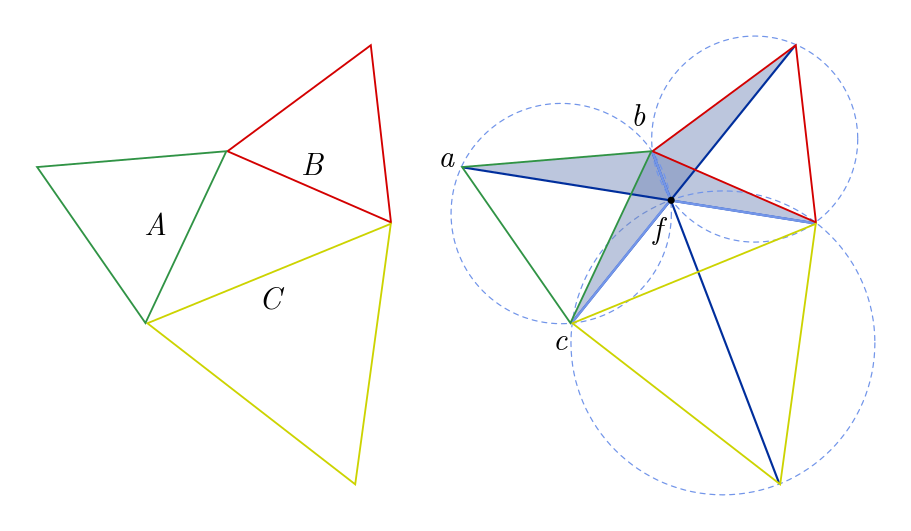Figure 18. Constructing the Fermat-Torricelli point.

Consider a triangle with sides $A, B, C$, displayed in Figure 18. On each side, attach equilateral triangles (green, red, yellow) of the corresponding side length. Draw lines from the outer corners of these attached equilateral triangles to the opposite corner of our original triangle. Our goal is to show that the dark blue lines intersect at angles of $120^\circ$ at point $f$.

The plan is simple. First, draw circles which circumscribe each equilateral triangle (dotted blue lines in Figure 18). We want to prove these circles intersect at $f$ using the inscribed angle theorem.

(a) Show that the shaded triangles are congruent. Argue that, in consequence, the three blue lines do interesect at a single point.

(b) From part (a), argue that $\angle baf = \angle bcf$.

(c) Conclude from (b) and the inscribed angle theorem that $a, b, c, f$ lie on a circle.

(d) Since the triangle is equilateral, $\angle cab = 60^\circ$. Using the inscribed angle theorem once more, show that $\angle cfb = 120^\circ$. Repeating this argument for the remaining two triangles gives our result!

(e) What happens when one of the original triangle’s angles is $120^\circ$?

## 4. Soap bubbles

Humans are not the only players in the minimisation game. Nature also likes to minimise, and has far more power at her disposal than us mere mortals. While we struggle with tinkertoys, Nature seems to minimise networks as effortlessly as a child dropping a ball (and in fact, Nature is solving a minimisation problem here as well). We’ll start by thinking about soap bubble networks in general, and finish by describing soap bubble computers to help us find minimal networks.

### 4.1. Foams

Bubbles are formed when a film of liquid separates two volumes of air. Surface tension tries to pull the bubble surface taut in all directions, which results in the minimisation of area. A soap bubble is spherical, for instance, since this is the smallest surface containing a fixed volume of air. If we attach two plexiglass plates together and dip them in soapy water, a two-dimensional network of bubbles with vertical walls will form. A single bubble will now be a cylinder, rather than a sphere, as in Figure 18.Figure 19. A soap bubble in three and two dimensions.

Typically, bubbles will form closed loops and hence are not minimal networks in our sense, since we explicitly ruled out loops earlier. But bubbles are locally minimal, in the sense that surface tension will tug on bubble walls, and reconfigure small bubbles, until doing so cannot reduce the area. And the result we found earlier, that hubs have three equally separated spokes, is a local condition, since it applies in the small region around a node! This means that in bubble networks, the same property is true: bubble walls always meet in sets of three, separated by $120^\circ$. This even applies to curved bubble walls, since when you zoom in on a junction these curved walls look straight.

We can use this to explain why two-dimensional foams form hexagons! We don’t need any physics, just the result about hubs, some network theory, and a few reasonable assumptions about what happens as a foam network gets bigger. The main fact about network theory is Euler’s formula, governing the relationship between nodes $N$, edges $E$, and faces $F$ in a graph:

$N - E + F = 2.$

This relation is illustrated for a cube in Figure 20. Note that, for a graph, we count the whole region outside the network as a face! Exercise 15 guides you through a proof of this formula.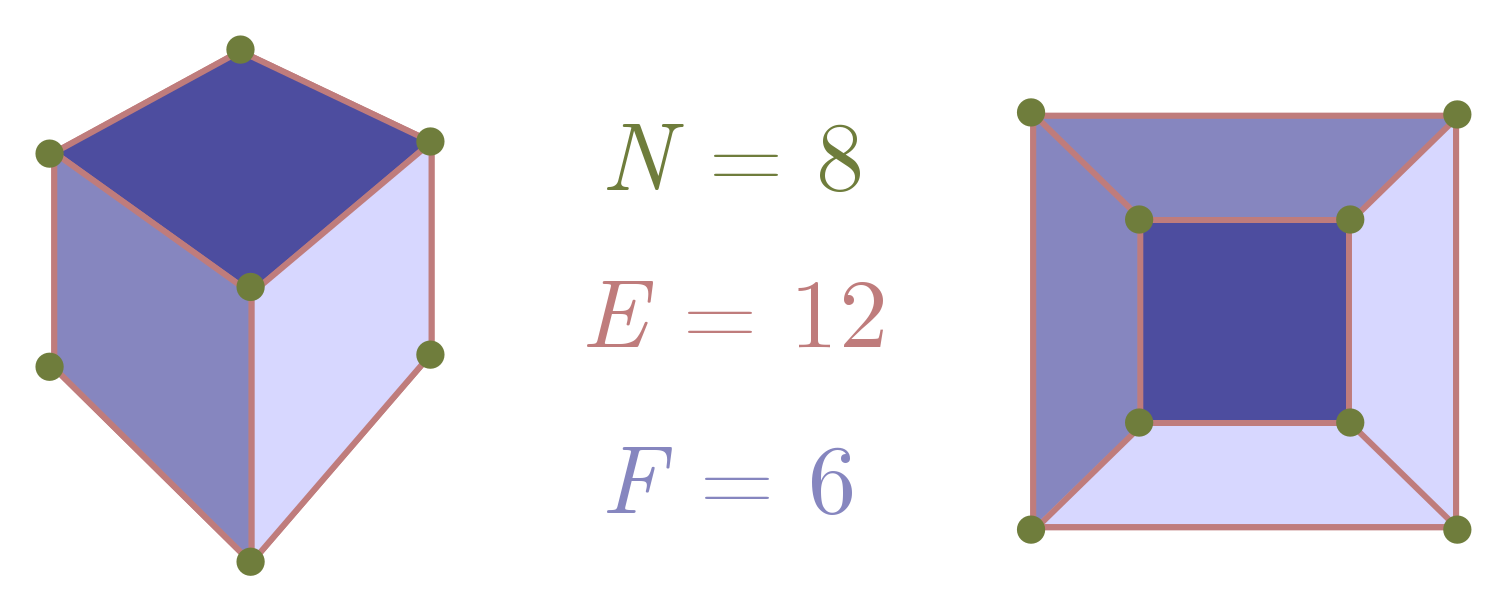Figure 20. Euler's formula illustrated for a cube.

Now, for a two-dimensional foam, there are no “external” nodes. Each node is a hub, with three edges, so

$2E = 3N,$

where we include the factor of $2$ as usual because of double counting. Putting this into Euler’s formula, we can eliminate $N$ and find a relation between the number of faces and number of edges:

$\frac{2}{3} E - E + F = 2 \quad \Longrightarrow \quad 3F - E = 6.$

As advertised, we can treat the external face a little differently. Let’s call the internal faces $F’$, so that $F = F’ + 1$. Rewriting in terms of $F’$, we get $3F’ - E = 3$.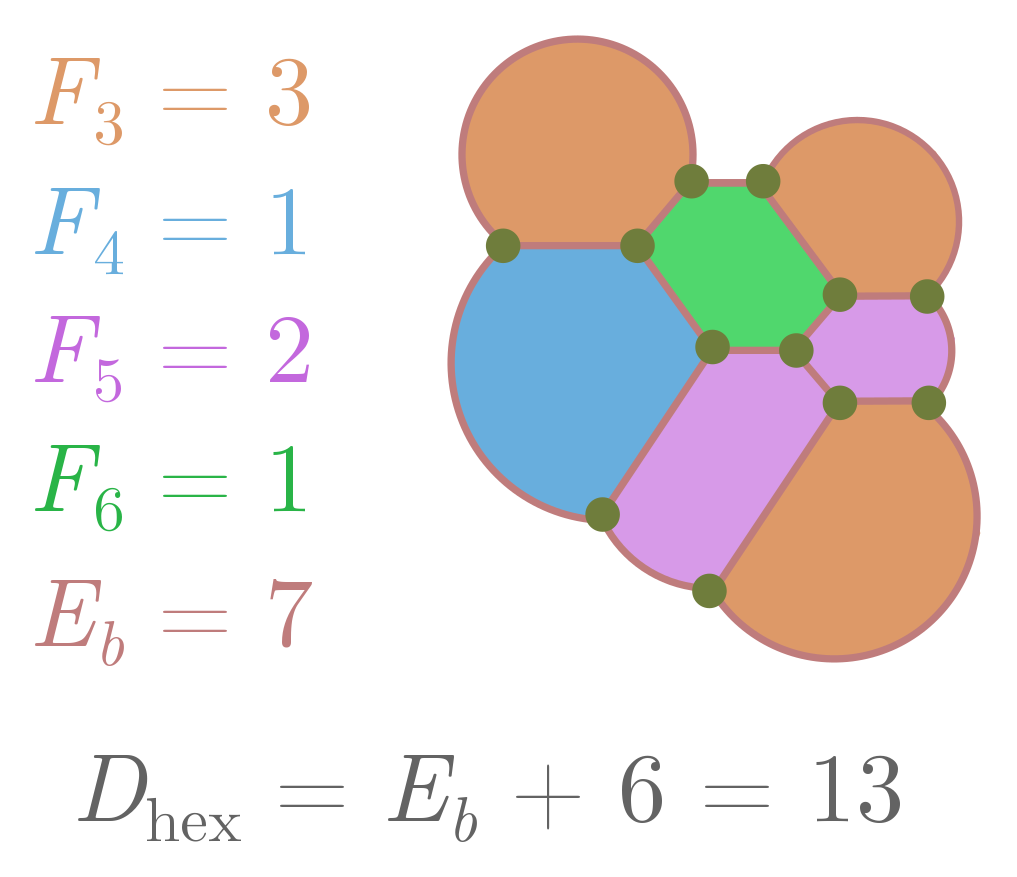Figure 21. An example calculation of the difference from hexagonality.

What has all this got to do with hexagons? Let’s introduce a number $F_s$ which counts the number of internal faces with $s$ sides, and $E_b$ stand for the number of edges of the outer face. Since we need at least one side to form a face, the total number of internal faces is

$F' = F_1 + F_2 + F_3 + \cdots \,.$

But since each edge is associated with two faces, we can also express edges in terms of these numbers:

$2E = E_b + 1 \cdot F_1 + 2 \cdot F_2 + \cdots + s\cdot F_s + \cdots \,.$

Since the $F_s$ only count the internal faces, and each external edge will be adjacent to one internal face. If we plug these expressions into $3F’ - E = 3$ and multiply everything by two, we finally get

$6 + E_b = 6F' - 2E + E_b = (6-1) \cdot F_1 + (6-2) \cdot F_2 + \cdots + (6-s)\cdot F_s + \cdots \,.$

On the LHS, we have a term involving the number of external edges $E_b$. On the RHS, we have the difference from hexagonality, which we’ll call $D_\text{hex}$. For a face with $s$ sides, $6-s$ tells you how different it is from a hexagon! So, more simply, we have

$D_\text{hex} = 6 + E_b.$

There are a few cute things we can learn from this expression. First of all, the LHS is positive, so $D_\text{hex} \geq 0$. The number of “small” faces $F_1, \ldots, F_5$ places a strong constraint on the number of “large” faces $F_7, F_8, \ldots$, and so on:

$5 \cdot F_1 + 4 \cdot F_2 + \cdots + 1\cdot F_5 \geq + 1 \cdot F_7 + 2\cdot F_8 + \cdots \,.$

So if I count the number of small faces, I can immediately figure out the maximum number of large faces!Figure 22. Approximate scaling of internal and external edges with total size L.

But our main goal was to show that foams are approximately hexagonal, so let’s proceed. Suppose the foam has overall size $\sim L$. Assuming bubbles have a typical size independent of $L$, the number of external edges $E_b \sim L$. For instance, if bubbles have average edge length $\ell$, independent of $L$, and we have a circular foam of radius $L$, then $E_b = (\pi/\ell)L$. It follows that, for large $L$,

$D_\text{hex} = 6 + E_b \sim L.$

The “density” of difference from hexagonality $d_\text{hex}$ is just the total difference divided by the area of the foam. This should scale as $L^2$, so that

$d_\text{hex} \sim \frac{D_\text{hex}}{L^2} \sim \frac{1}{L}.$

As the foam becomes larger, differences from hexagonality become increasingly rare! Thus, a typical bubble in a two-dimensional foam has six sides. Pictured below is a real foam. It consists almost entirely of hexagonal cells, just as our maths predicts!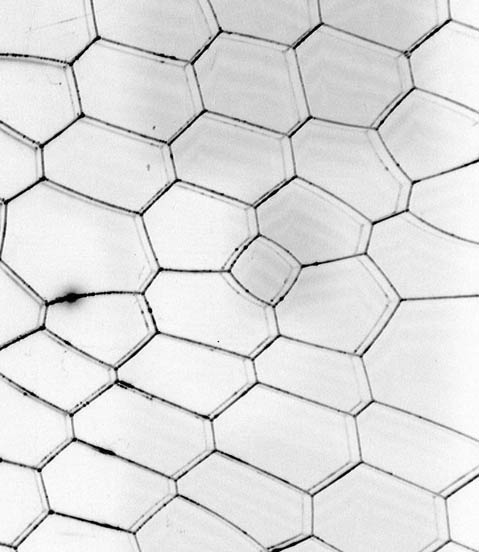A real two-dimensional foam. Klaus-Dieter Keller, Wikimedia Commons.

Exercise 14 (bubble blobs). A “bubble blob” is a set of contiguous bubbles in a (two-dimensional) foam. Let $E_o$ denote the number of edges extending outward from the boundary, and $E_i$ the number extending inward.

(a) Explain why the difference from hexagonality is now

$D_\text{hex} = 6 + E_i - E_o.$

(b) Repeat the scaling argument above, and conclude that in a large blob, departures from hexagonality become harder and harder to find.

Exercise 15 (Euler’s formula). Our goal here will be to prove Euler’s famous formula:

$N - E + F = \chi = 2,$

where $N$ is the number of nodes, $E$ the number of edges, and $F$ the number of faces, in a network where edges do not cross. We have also defined $\chi = N - E + F$, called the Euler characteristic, for notational convenience. Although we are discussing this in the context of graphs, it holds in general for three-dimensional polyhedra like cubes and dodecahedra. In fact, the two are equivalent, since we can always flatten a polyhedron to get its net, which is a network.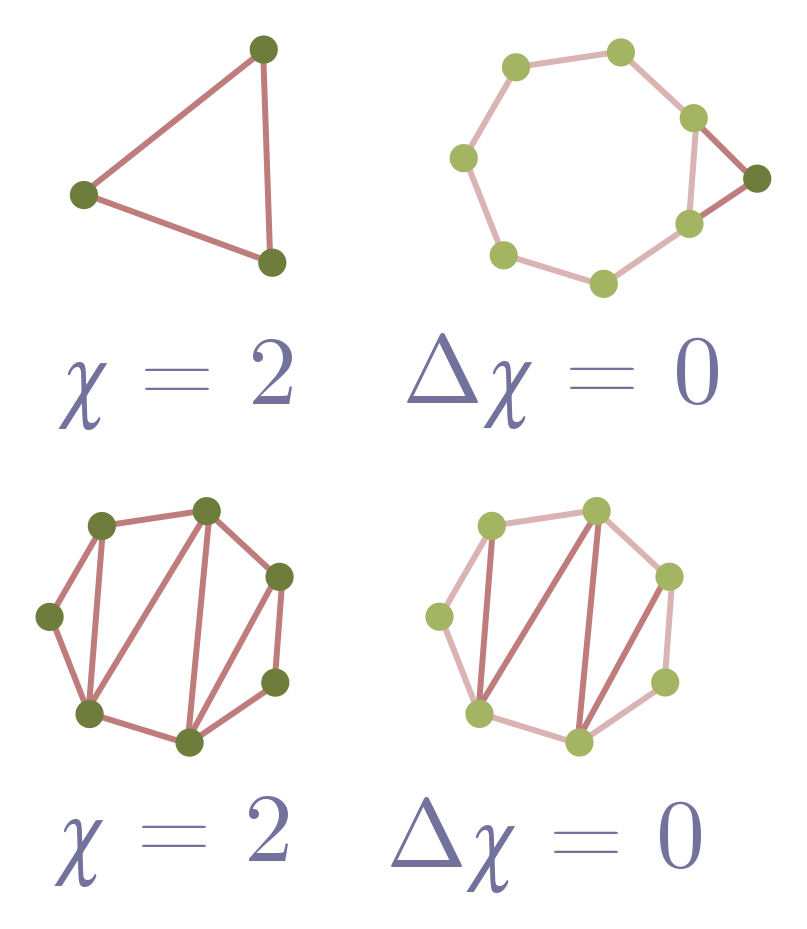Figure 23. Steps (a), (b), (c) and (e) illustrated.

First, we will establish Euler’s formula for networks made out of triangles.

(a) Show that a lone triangle in the plane obeys Euler’s formula. Remember, we count the area outside the triangle as a face!

(b) Suppose I have a network which obeys Euler’s formula. Add a triangle (two edges and a node) to an external edge, and explain why the Euler characteristic doesn’t change, $\Delta \chi = 0$. Conclude that the new network obeys Euler’s formula.

(c) Explain why any network composed entirely of triangles obeys Euler’s formula.

Now, we can generalise to any network without crossings.

(d) Consider a face, i.e. loop, in such a network. Describe a procedure to add edges so that the face is split into triangles.

(e) Show that, after your procedure in part (d), $\Delta \chi = 0$.

(f) Combine your results to conclude that any network without crossings obeys $\chi = 2$.

### 4.2. Hyperbolic honeycomb*

In this section, we find an interesting connection between honeycombs and hyperbolic space. The material is slightly more advanced than the rest of the post, as indicated by the asterisk.

First, you may have wondered if the hexagonality of foams is related to the fact that bees build honeycombs in a hexagonal lattice. It is! Bees have a clear evolutionary reason to minimise the amount of wax used. Darwin discusses the hive-making instinct and its relation to fitness in his Origin of Species:

That motive power of the process of natural selection having been economy of wax; that individual swarm that wasted least honey in the secretion of wax, having succeeded best.

For honeycomb walls to be locally minimal, they must obey the $120^\circ$ rule. If we also require our honeycomb cells to be equal size, then a natural guess at the optimal arrangement is the hexagonal lattice, and indeed, this is the only regular tesselation of the plane which satisfies the $120^\circ$ rule. The honeycomb conjecture states the hexagonal lattice is the globally minimal solution. It turns out to be hard to verify this guess, since you need to somehow check all possible irregular tilings as well, but in 1999, it became the honeycomb theorem after Thomas Hales gave a formal proof. We will consider the three-dimensional analogue of the honeycomb lattice in a later section.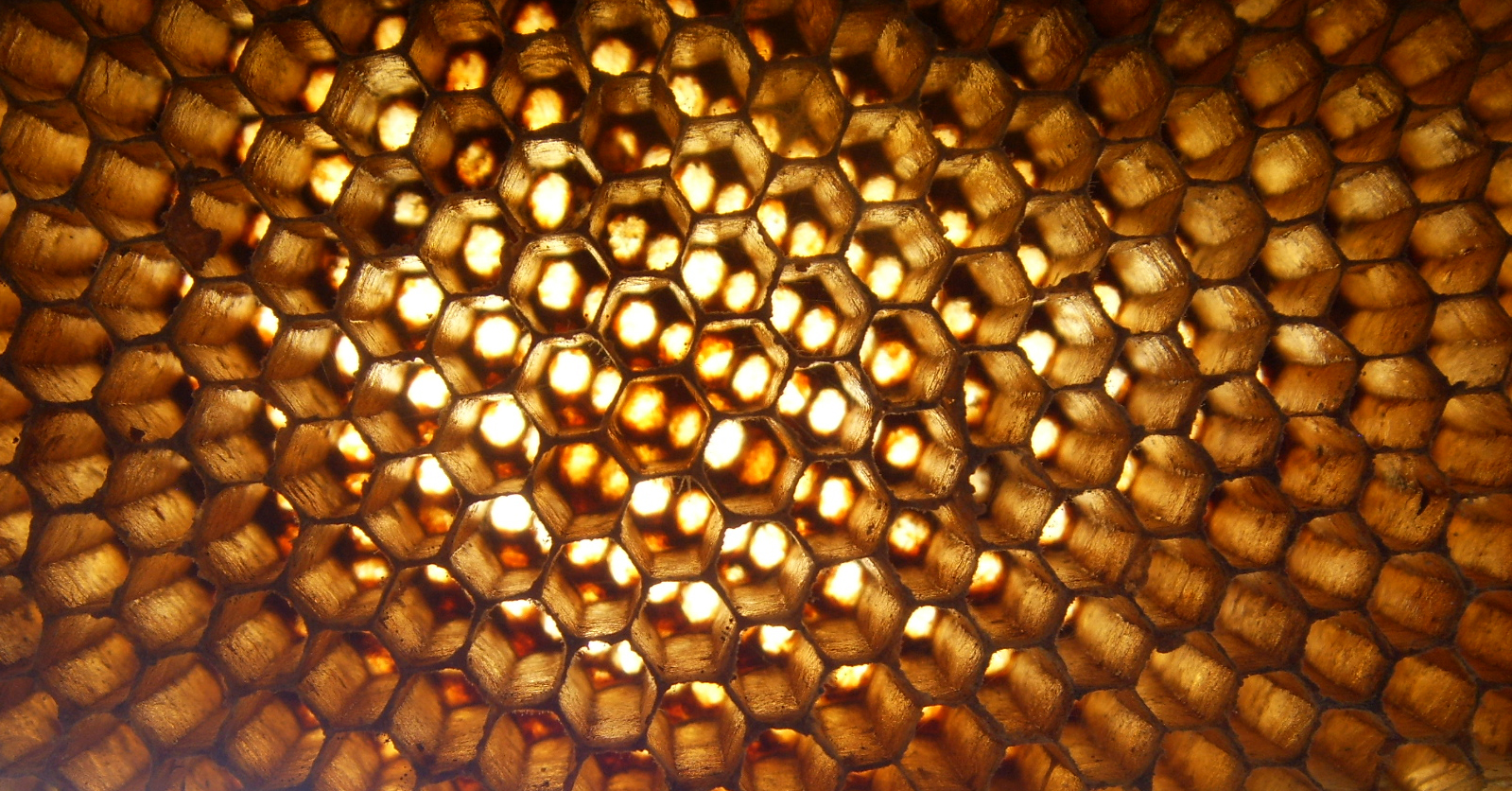An abandoned beehive. Sean Hoyland, Wikimedia Commons.

Our next point concerns the scaling assumptions in the previous section. We stated that if a two-dimensional foam has size $\sim L$, then its boundary will also scale as $L$, which is the case for e.g. a circle in the plane. We have secretly made a big assumption here: the foam lives on a flat plane. But the foam could live on a curved surface, such as a saddle or a sphere. We will focus on the saddle.

Although they seem obscure, saddle-shaped surfaces are important and beloved of mathematicians, who study them under the guise of the hyperbolic plane. In the hyperbolic plane, the perimeter of a foam is huge: it scales as $L^2$ rather than $L$! Our earlier claim that $D_\text{hex} = 6 + E_b$ is still true, but now $D_\text{hex} \sim L^2$. As a consequence, the average departure from hexagonality can now be some nonzero number:

$d_\text{hex} = \frac{D_\text{hex}}{L^2} \sim 1.$

There are hyperbolic foams made of heptagons ($d_\text{hex}=1$), octagons ($d_\text{hex}=2$), and even nonagons ($d_\text{hex}=3$). Here is a picture of my personal favourite, the heptagonal tiling: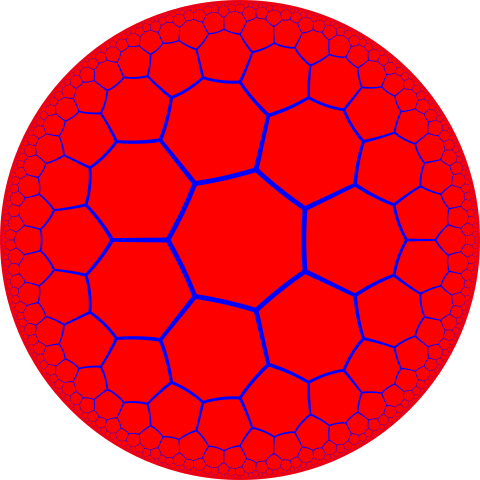A hyperbolic foam made of heptagons. Parcly Taxel, Wikimedia Commons.

The heptagonal tiling is not only beautiful, but useful for bees living in hyperbolic space. The weird properties of saddles mean that the optimal foam depends on the size of the cells, but in November 2019, a group of undergraduates proved that for compartments of size $\pi/3$, the heptagonal tiling is optimal, at least among regular tilings. The “hyperbolic honeycomb conjecture” — that this is optimal among all tilings with cells of area $\pi/3$, including the irregular ones — remains open. Perhaps we should breed some hyperbolic bees, and come back in a few thousand years!

### 4.3. Computing with bubbles

Soap bubbles find local minima quickly because of the laws of physics. Can we somehow “hack” soap bubbles, and turn them into analogue computers which find Steiner trees? Sort of. Computer scientist Scott Aaronson has persuasively argued that hard problems (such as finding Steiner trees) cannot be solved quickly, in the general case, by any physical mechanism. So as a matter of physical principle, we should not expect soap bubbles to quickly find arbitrary minimal networks.

But this doesn’t prevent soap bubbles from giving quick and correct solutions to some problems, nor does it forbid them from approximating the answer. Indeed, there are quick approximation algorithms for regular computers, so we shouldn’t be surprised if soap bubbles can do the same. So, can we quickly (and perhaps approximately) find some minimal networks by dipping them in soapy water? The answer is yes!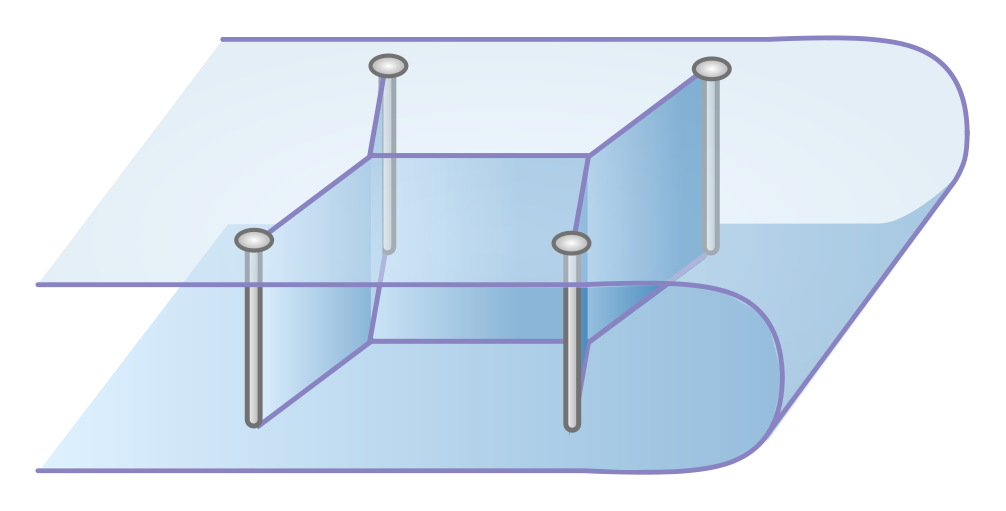Figure 24. Soap bubbles computing a minimal network.

The key is to give the bubble walls something to hold onto (Figure 24). In a foam, they can only hold onto themselves, and we get the non-crossing bubble networks described above. But if we drill some screws through the plexiglass, these will act like fixed nodes, and walls can form between these screws and hubs arising from the junction of three bubble walls.

This analogue computer isn’t perfect, since the soap bubbles can converge on a locally minimal tinkertoy which connects the screws, but is not globally minimal. But it really is a lucky dip, and you can sometimes strike the true minimum, particularly for smaller problems where only one tinkertoy works. See this video for a demonstration.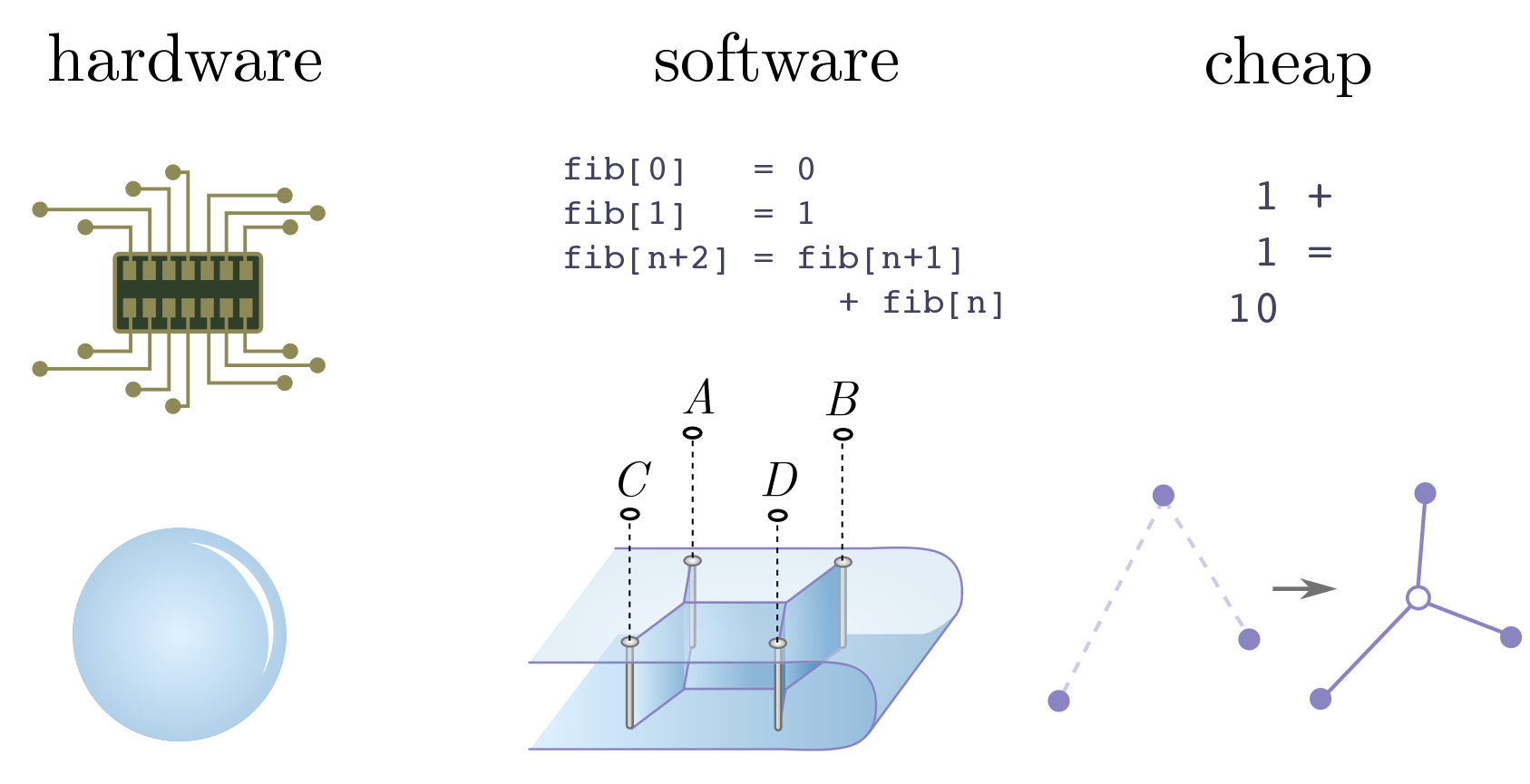Figure 25. Hardware, software and cheap operations.

Soap bubbles do not magically solve problems thought to be impossible. But they do demonstrate strikingly that physical objects are computers, and the laws of physics do computational work. To quote Rolf Landauer,

Information is physical.

We can push this obervation further, but for our purposes, let’s just consider the distinction between hardware and software. Hardware is the physical machinery the computer is built on. It tells us which operations are basic, and therefore computationally cheap. Software is a layer of instruction and abstraction above the hardware, telling the computer how to represent data and manipulate it to do useful things.

In the soap bubble computer, the hardware is clearly the bubbles themselves, and local area minimisation a cheap operation by virtue of the laws of physics. The software is nothing more complicated than drilling screws, dipping, and waiting for bubbles to form. But this is just the tip of the physical computer iceberg! The last few years has seen the advent of quantum computing, with computers which can hopefully leverage the weirdness of quantum mechanics to outperform non-quantum computers. Perhaps more surprisingly, there is a sense in which black holes, DNA, evolutin (see the previous section) and animal brains are all special-purpose analogue computers, just like the soap bubbles. There are more computers in heaven and earth than are dreamt of in our philosophy!

Exercise 16 (trains and soap bubbles). As advertised above, the first mathematician to consider minimal networks on four points was Gauss. In an 1836 letter to his friend, the astronomer Schuhmacher, Gauss asked:

How can a railway network of minimal length which connects the four German cities Bremen, Harburg, Hannover, and Braunschweig be created?

The cities are drawn in Figure 26: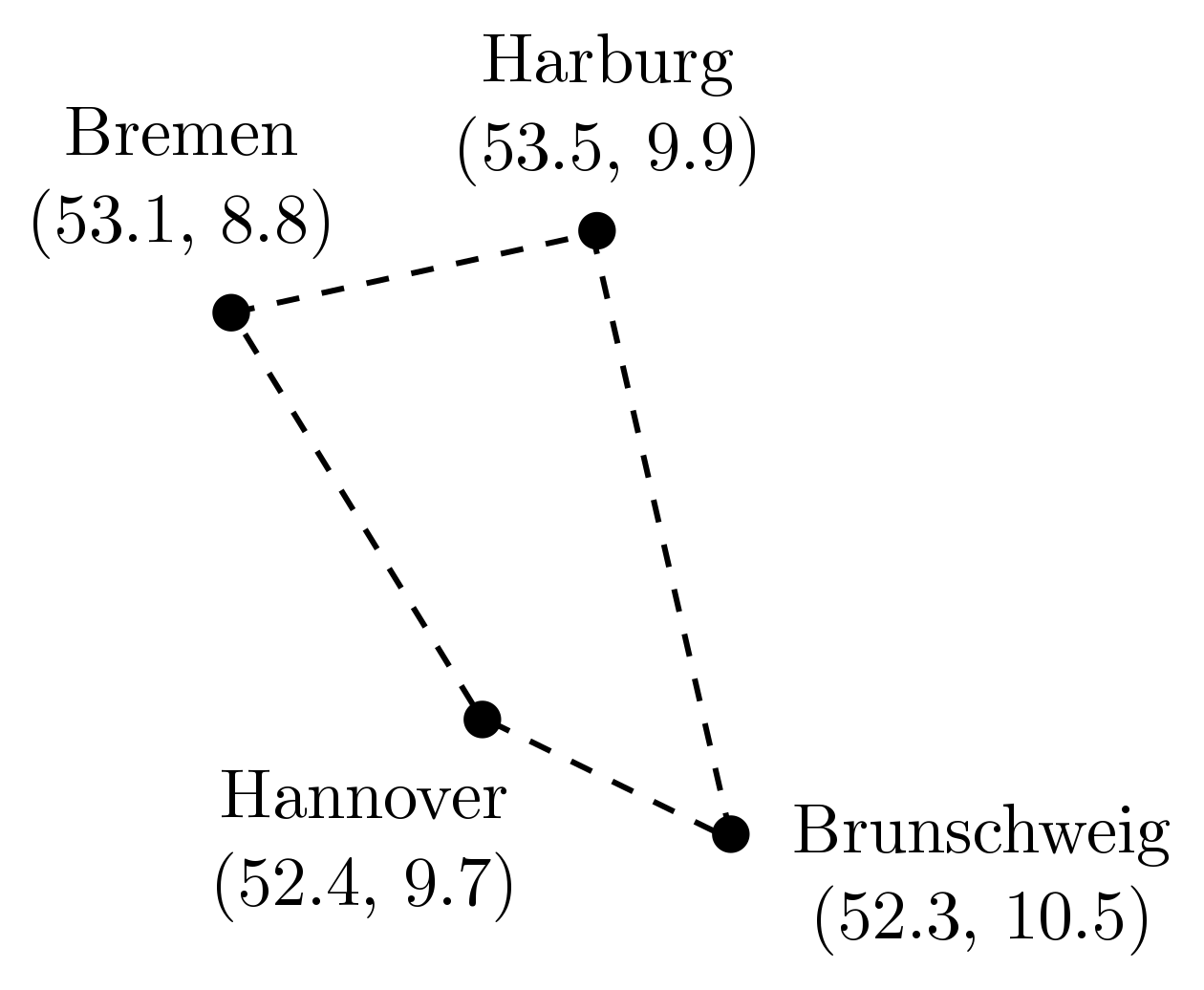Figure 26. German cities to be connected by rail, with GPS coordinates.

Your task is simple: build a soap bubble computer, and use it solve the original rail network problem! Figure 26 gives the GPS coordinates, which you may find useful in placing your screws.

### 4.4. Plateau’s laws and infinite foams*

Edit: Section added April 8, 2020. Pictures to come!

We’ve described the rules obeyed by two-dimensional foams. But in real life, foams are three-dimensional, and it behoves us to say a little about them. We will do this in the form of exercises.

A two-dimensional foam has areas separated by a locally minimal network, but in three dimensions, bubble cells are volumes separated by a locally minimal surface. Two-dimensional foams obey the $120^\circ$ rule, while three-dimensional foams obey a generalisation called Plateau’s laws. These are named after the Belgian mathematician Joseph Plateau (1801-1883), who guessed them by assiduously observing soap bubbles!

Here are Plateau’s first three laws:

1. The faces in a soap film are smooth, i.e. no kinks.
2. Any face has constant mean curvature.
3. Faces always meet three at a time, separated by $120^\circ$.

The edge shared between three faces is called a Plateau border. Constant mean curvature is a technical term, but simply put, means that if we make a straight incision through the soap film, the resulting curve is a straight line or the arc of a circle. This does not mean that bubble walls are flat or spherical; they can also saddle-shaped, like the hyperbolic plane we mentioned above.

Exercise 17. Let’s now take a cross-section $A$ which “cuts through” every face and every Plateau border of the foam, forming a network where edges correspond to bubble faces and points to Plateau borders.

(a) Show that if $A$ is perpendicular to a Plateau border, edges meet at the corresponding point at angles of $120^\circ$.

(b) If $A$ is not perpendicular to a Plateau border, prove the edges do not meet at $120^\circ$.

(c) Explain why our earlier formula $D_\text{hex} = 6 + E_b$ holds for the cross-section, despite the results of the previous exercise. Under the scaling assumptions we made above, faces in $A$ tend to be hexagonal.

Exercise 18. The first three laws don’t tell us what happens when Plateau borders meet. To motivate Plateau’s final law, let’s revisit the $120^\circ$ law for hubs and spokes.

(a) Suppose Plateau’s first law applies to a network, i.e. no kinks in an edge. Explain why at least three edges must meet at a vertex.

(b) Show that separation by $120^\circ$ is the most symmetric arrangement of three edges.

Our rule for hubs and spokes in minimal networks therefore follows from a different set of assumptions: first, the minimal number of edges meets at a node, and second, the edges are arranged symmetrically. Generalising these principles leads to Plateau’s fourth law. From now on, “vertices” refers to the foam, and not the cross-section. (We can actually use these assumptions to describe higher-dimensional foams, but three dimensions is more than enough!)

Consider a configuration of Plateau borders meeting a vertex of the foam.

(c) From Plateau’s first law, explain why at least four Plateau borders meet at a vertex.

(d) If four borders meet at a vertex, what is the most symmetric arrangement?

(e) Show that, for part (b), the borders are separated by angle $\theta = \cos^{-1}(-1/3)$.

This leads to Plateau’s fourth law:

4. Four Plateau borders meet at a vertex, separated by the angle $\theta \approx 109.5^\circ$.

In the last section, we were able to hack two-dimensional foams by inserting screws to act as as fixed points. Similarly, we can hack a three-dimensional foam by adding fixed boundaries made out of wire and immersing in soapy water. A bubble blower is a kind of computer too! Generalised bubbles blowers are used for most applications of minimal surfaces, for instance tensile structures in architecture.

But instead of fixing a boundary, we can fix the volume of a bubble, i.e. require that it enclose a given volume of air. The simplest example is a single bubble. The only smooth, constant mean curvature surface enclosing a finite volume is the sphere (an intuitive but mathematically tricky statement to prove). It is clear from blowing bubbles — which enclose precisely the volume of air you blow into them — that nature opts for this solution, and it turns out that the sphere is not only locally minimal, but globally minimal as well! This deep mathematical result is called the isoperimetric inequality. While hard to prove, it is easy to guess from the bubble blower!

Exercise 19. Consider two bubbles of fixed volume $V$. Assuming that extra bubbles increase the surface area, use Plateau’s law to determine the shape of the minimal surface. You may also assume that the face between bubbles is flat. (Mathematicians proved this “double bubble” configuration was globally minimal in 2002.)

Exercise 20. Using similar techniques to our hexagonality argument, we can show that faces of three-dimensional bubbles have less than $6$ sides on average.

(a) Prove the three-dimensional analogue of Euler’s formula,

$N - E + F = C,$

where $C$ is the number of bubble cells. (As with faces, we count the region external to the bubbles as a single cell.) Hint. Consider removing $C - 2$ faces.

(b) From Plateau’s fourth law, argue that $E = 2N$.

(c) Let $F_\text{avg}$ denote the average number of faces per cell and $E_\text{avg}$ the average number of edges per cell. Show that

$F_\text{avg} = \frac{2F}{C}, \quad E_\text{avg} = \frac{3E}{C}.$

Hint. You will need Plateau’s third law for the second identity.

(d) Combine (a), (b) and (c) to deduce the relation between average number of edges and average number of faces:

$3F_\text{avg} - E_\text{avg} = 6.$

This is analogous to the result we proved for two-dimensional foams, $3F - E = 6$.

(e) Let $e_\text{avg}$ be the average number of edges per face. Derive the relation

$F_\text{avg} = \frac{12}{6- e_\text{avg}}.$

(f) Conclude that, unlike a two-dimensional foam, the average number of edges per bubble face is strictly less than $6$. Why is this consistent with the result that cross-sections of the foam tend to be hexagonal?

Exercise 21. In the previous exercise, we introduced a relation between the average number of faces of a cell, edges of a cell, and edges of a face. Since we are discussing averages, they continue to make sense even if the foam is infinite! The simplest infinite foams are those in which each bubble is the same, so the shape of the bubbles forms a space-filling tessellation.

As a warm-up to studying tessellations of three-dimensional space, we’ll study tessellations of the plane. The simplest tessellations are by regular polygons, and as we will see, there are only three possibilities.

(a) Prove that the internal angle of a regular $d$-gon (in radians) is

$\theta = \pi \left(\frac{d-2}{d}\right).$

(b) Assume that the regular polygons are tiled so they meet at a vertex. Explain why we must have $2\pi = n\theta$ for some whole number $n$, where $\theta$ is measured in radians.

(c) Conclude that the square, triangle and hexagon are the only possibilities, and work out $n$ (the number meeting at a vertex) for each.

(d) Show that only the hexagonal lattice can describe a two-dimensional foam.

As discussed in a previous section, this is connected to the fact that the hexagonal lattice is the most efficient way to separate cells of equal size. We can use these planar tessellations to tessellate three-dimensional space as well, but using prisms: boxes, triangular prisms and hexagonal prisms. Can these form three-dimensional foams?

(e) Check that the prisms satisfy the requirements of Exercise 20(d).

(f) Show that, nevertheless, a prism-based tessellation cannot satisfy Plateau’s laws.

Exercise 22. We have ruled out prisms as the basis for an infinite, three-dimensional foam of equally-sized bubbles. We next consider regular polyhedra, or Platonic solids. There are only five regular polyhedra: the cube, tetrahedron, octahedron, dodecahedron and icosahedron. A cube is a type of square prism, so the previous exercise rules this out. We now want to see if any of the remaining solids can fill space.

We’ll focus on what happens a corner. In the plane, we need to gather corners together so they subtend a full revolution of $2\pi$ radians. In three dimensions, we must gather corners together so that they subtend a full “solid revolution” of $4\pi$ “solid radians” or steradians. The solid vertex angles for the Platonic solids (except the cube) are as follows:

\begin{align*} \Omega_\text{tetrahedron} & = \cos^{-1}(23/27) \\ \Omega_\text{octahedron} & = 4\sin^{-1}(1/3) \\ \Omega_\text{dodecahedron}& =\pi - \tan^{-1}(2/11)\\ \Omega_\text{icosahedron} & = 2\pi - 5\sin^{-1}(2/3). \end{align*}

For simplicity, we’ll assume that corners of a cell meet corners of adjacent cells, rather than edges or faces. (The general case follows from similar, but more finnicky, reasoning.)

(a) Argue that, for a Platonic solid to tessellate, it must have vertex angle $\Omega = 4\pi/n$ for some integer $n$, assuming only corners meet.

(b) Using a symbolic algebra package, show that no Platonic solids (other than the cube) have this property.

Thus, the only Platonic solid which can tessellate is the cube. We have already shown this doesn’t make foams, so we must seek bubbles elsewhere!

Looking beyond Platonic solids, the next simplest polyhedra are the “semi-regular” Archimedean and Catalan solids. There are $26$ of these altogether, so I won’t try to describe them all. We saw in the previous exercise that, even for Platonic solids, working out which ones can tessellate takes effort, so we’ll just skip straight to the answer. Only two can fill space: the rhombic dodecahedron (so called because it has twelve rhombic faces) and the truncated octahedron (obtained by snipping off, or truncating, the corners of an octahedron).

We can also extrude each face of the rhombic dodecahedron to a pyramidal point. The result is the stellated rhombic dodecahedron, aka “Escher’s solid” after M. C. Escher’s marvellous lithograph Waterfall. (Escher’s solid is a stellation which also tessellates space, so perhaps we should call it the “testellation”.) To summarise, we can fill space with cells of the following type:

• the rhombic dodecahedron, with twelve rhombic faces;
• Escher’s solid, with $48$ triangular faces; and
• the truncated octahedron, with eight hexagonal faces and six squares.

Only one of these tessellations is consistent with Exercise 20, and can therefore describe a foam. It is called the Kelvin structure.

(c) Which one is it?

Lord Kelvin (1824-1907) conjectured that the Kelvin structure was the most efficient way to separate equal volume cells. This is just a three-dimensional version of the honeycomb conjecture stated above. If correct, it’s what four-dimensional bees would store their honey in! And like the honeycomb, the Kelvin structure is the only “simple” tessellation which is locally minimal. (Note that, strictly speaking, we need to bend the edges a little to ensure they meet at $\theta \approx 109.5^\circ$.)

But there are reasons to be cautious. Our little catalogue of tessellations suggests that the options are much richer in three dimensions than in two. Perhaps we shouldn’t be so quick to dismiss the possibility that some other configuration, with an odd shape, or two or more cell types of the same volume, beats the Kelvin structure for efficiency. And indeed, in 1993, more than 100 years after Kelvin made his bold conjecture, Weaire and Phelan improved on the Kelvin structure by weaving together two funny-shaped cells of equal volume. (It is unknown whether this is globally optimal.)

Exercise 23. The Weaire-Phelan structure uses two different cells of equal volume:

• an irregular dodecahedron $C_1$, with twelve hexagonal faces; and
• a “tetrakaidecahedron” $C_2$ of two hexagonal and twelve pentagonal faces.

What ratio between $C_1$ and $C_2$ is needed for consistency with Exercise 20?

Like blowing bubbles to check the isoperimetric inequality, Weaire and Phelan found their solution by performing computer simulations of foam. Careful experiments with soap film also reproduce the structure, and it can be observed in certain crystalline compounds. Once again, the laws of physics provide natural computers for solving deep mathematical problems! But as with soap films, these computers are far from perfect. Weaire discusses some of the experimental difficulties of obtaining the Weaire-Phelan structure here. In many cases, Nature prefers the Kelvin structure!

It’s wonderful that something as ordinary-seeming as a soap bubble could embody such a rich interrelationship between physics, mathematics and computing. To quote Charles Boys’ classic book,

I hope that none of you are yet tired of playing with bubbles, because as I hope we shall see, there is more in a common bubble that those who have only played with them generally imagine.

There is more in playing with them than generally imagined as well.

#### Acknowledgments

I’d like to thank Rafael Haenel, Pedro Lopes and Haris Amiri for feedback, and Rafael particularly for his encouragement. Some of this material was tested at the UBC Physics Circle, as part of a workshop run by Geering Up and Diversifying Talent in Quantum Computing. I would like to thank the students for their participation and feedback.